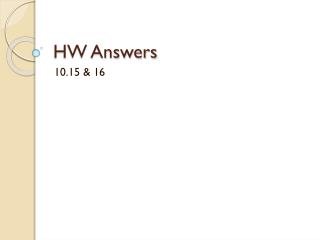# HW Answers - PowerPoint PPT PresentationDownload PresentationDownload Presentation- - - - - - - - - - - - - - - - - - - - - - - - - - - E N D - - - - - - - - - - - - - - - - - - - - - - - - - - -
##### Presentation Transcript

1. HW Answers 10.15 & 16

2. Catalyst • In the following chemical reaction, hydrogen peroxide is broken down into water and oxygen. • __H2O2→ __H2O + __O2 • Balance the chemical equation. • Is this a decomposition or synthesis reaction? • What is the mole ratio of water to oxygen? • What is the mole ratio of hydrogen peroxide to water?

3. Objectives and Agenda • Objective: I can create mole ratios from a balanced equation and make mole to mole conversions. • Agenda: • Catalyst • Learning target logs • Mole ratio and conversion practice

4. Learning Target Logs & Portfolios • We will be tracking progress towards standards mastery on learning target logs for Unit 4. • Please put your name on the learning target log. • We will go through how to fill it out together.

5. Assignment • Read p. 302 – 305; Do practice problems p. 304 #1 & 2, p. 311 #1, 4(a), 6. • For more practice: • Pp. 329-330 # 8, 9, 21, 22

6. p. 304 #1 • Calculate the amounts requested if 1.34 mol H2O2completely react according to the following equation. 2H2O2 → 2H2O + O2 • Moles of Oxygen (O2) formed? • Converting from mol H2O2to mol O2 1.34 mol H2O2 1 mol O2___ 2 molH2O2 0.67 mol O2 = X This is the amount given in the question. This is the mole ratio from the balanced equation.

7. p. 304 #1 • Calculate the amounts requested if 1.34 mol H2O2completely react according to the following equation. 2H2O2 → 2H2O + O2 b. moles of water (H2O) formed? • Converting from mol H2O2to molH2O 1.34 mol H2O2 2 molH2O 2 molH2O2 1.34 mol H2O = X

8. p. 304 #2 • Calculate the amounts requested if 3.30 mol Fe2O3completely react according to the following equation. Fe2O3 + 2 Al→ 2 Fe + Al2O3 a. moles of aluminum (Al) needed? • Converting from mol Fe2O3to molAl 3.30 mol Fe2O3 2 molAl____ 1 molFe2O3 6.60 mol Al = X

9. p. 304 #2 • Calculate the amounts requested if 3.30 mol Fe2O3completely react according to the following equation. Fe2O3 + 2 Al→ 2 Fe + Al2O3 b. moles of iron (Fe) formed? • Converting from mol Fe2O3to mol Fe 3.30 mol Fe2O3 2 mol Fe___ 1 molFe2O3 6.60 mol Fe = X

10. p. 304 #2 • Calculate the amounts requested if 3.30 mol Fe2O3completely react according to the following equation. Fe2O3 + 2 Al→ 2 Fe + Al2O3 c. moles of aluminum oxide (Al2O3) formed? • Converting from mol Fe2O3to molAl2O3 3.30 mol Fe2O3 1 molAl2O3 1 molFe2O3 3.30 molAl2O3 = X

11. p. 311 #1, 4(a) • The mole ratio is the conversion factor that is present in almost all stoichiometry calculations. • (a) How many moles of BrClform when 2.74 mol Cl2react with excess Br2? Br2 + Cl2→ 2BrCl 2.74 mol Cl2 2 molBrCl 1 mol Cl2 5.48 molBrCl = X

12. p. 311 #6 A: In order to solve stoichiometry problems we have to use moles and not just convert from mass to mass because the balanced chemical equation expresses the ratios of reactants and products according to moles, not grams.